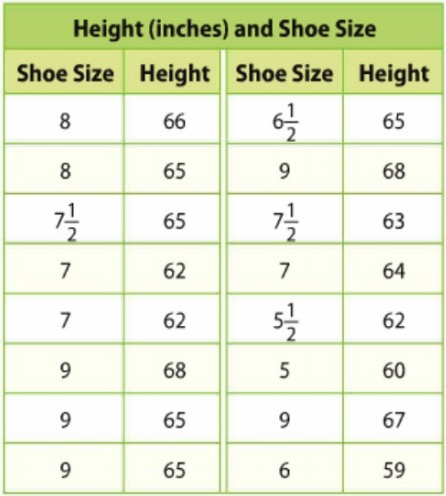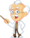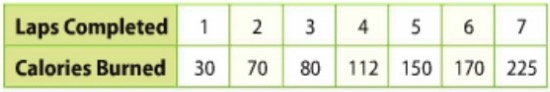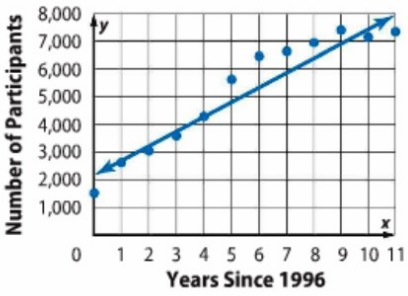Homework Explained - Math Practice 101Dear guest, you are not a registered member. As a guest, you only have read-only access to our books, tests and other practice materials.

As a registered member you can:

 Independent Practice Question 1 The results of a survey about women's shoe sizes and heights are shown.a. Construct a scatter plot for the data. Then draw and assess a line that best represents the data. Type below:(show solution) Question 1 b. Use the line of best fit to make a conjecture about the height of a female who wears a size 5 shoe. Type below:(show solution) Question 2 The table shows the number of Calories burned when walking laps around a track.a. Construct a scatter plot for the data. Then draw and assess a line that best represents the data. Type below:(show solution) Question 2 b. Write an equation for the line of best fit. Use the equation to make a conjecture about the number of Calories burned if someone walks 15 laps. Type below:(show solution) Question 3 The scatter plot shows the number of girls who participate in ince hockey.a. Write an equation in slope-intercept form for the line of best fit that is drawn, and interpret the slope and y-intercept. Type below:(show solution) Question 3 b. Use the equation to make a conjecture about the number of girls that will participate in ice hockey in 2020. Type below:(show solution)